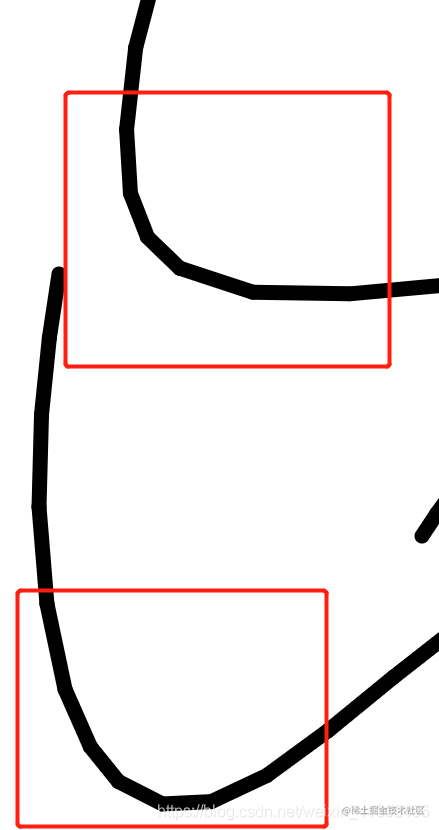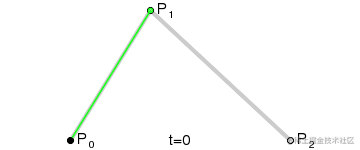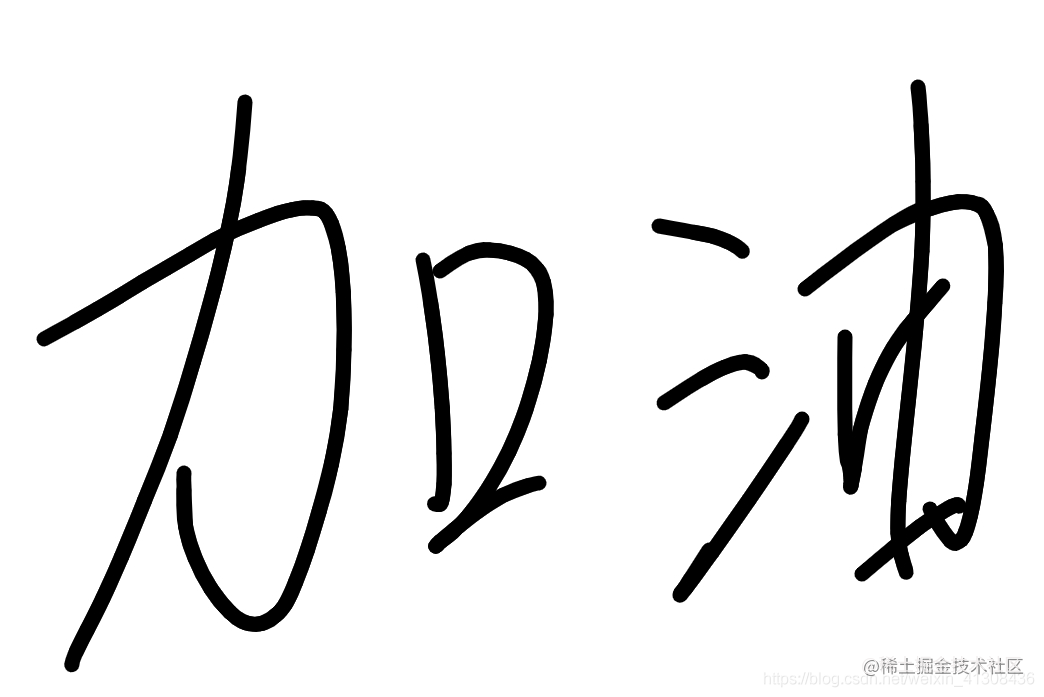# 反向求二次贝塞尔曲线的控制点，用贝塞尔曲线编写签名板，实现平滑的笔条，无菱角

## 1、最简单的实现方法就是用以下的接口

``````this.ctx.moveTo();
this.ctx.lineTo()
``````## 2.解决方案：二次贝塞尔曲线`````` P0（x1,y1）,P2(x2,y2), Pc(cx,cy)起点，终点，控制点
``````

``````cx = (x - (Math.pow(1 - t, 2) * x1) - Math.pow(t, 2) * x2) / (2 * t * (1 - t))
cy = (y - (Math.pow(1 - t, 2) * y1) - Math.pow(t, 2) * y2) / (2 * t * (1 - t))
t[0-1]
``````